Up | ObjectPosition3D - 105 | CreatePointCloud3D - 109 | ReferenceOnPlane3D - 143 | SphereFit3D - 141 | ModifyReference - 131 | ReferenceDiff3D - 126 | MapTo3D - 125 | ExtractMap3D - 122 | PlaneFit3D - 121 | CylinderFit3D - 112 | ChangeReference3D - 96 | Locate3D - 97 | MoveReference3D - 107 | ExternalReference3D - 95 | Calibrator3D - 155 | LineFromPoints3D - 98 | ReferenceFromPoints3D - 99 | Angle3D - 100 | NearestPointOnLine3D - 101 | ExternalPoint3D - 103 | ExternalLine3D - 104 | Point2Dfrom3D - 106 | GlobalPosition3D - 108 | Point3Dfrom2D - 115 | PointFromLines3D - 116 | ReferenceFromLines3D - 117 | LaserProfiler3D - 166 | NearestPointOnPlane3D - 118 | ChangePointReference3D - 119 | ChangeLineReference3D - 120 | PointOnLine3D - 130 | MoveLine3D - 138 | MovePoint3D - 137 | PlanePlaneIntersect3D - 139 | PlaneLineIntersect3D - 142 | Combine3D- 161 Commands | Shortcuts | Release Notes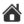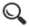Locate3D - 97

The Locate3D tool locates objects in three coordinates: x, y and z.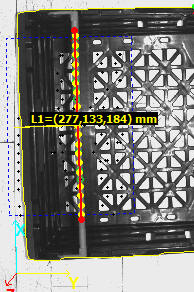STC-0038 FitLine3D locates a line based on the Locate3D matching the output from two 2D-lines.

The tool requires the following information from two, three or four cameras:

• 3D CameraCalibration - ExternalReference3D
• x,y from a set of corresponding points in multiple images

Powerful features for Locate3D are:

• Matches multiple unsorted 2D features points into a valid 3D feature set
• use option Automatically connect matching points
• 2D feature points can be classed to improve matching speed and accuracy
• Use z - range constraints to remove 3D folding challenges

Test profile

Here is a good 3DStereoVisionDemo profile for the Locate3D tool. It contains a set of images to understand how to use Locate3D.

The  Mono3D test profile is a companion profile that does the same function with the MonoPose3D tool.

Multiple demonstration profiles are available in the Scorpion Vision Installer.

Setup

3D Reference tools (one per camera image) - 3D Reference system selection

• An ExternalReference3D tool instance per camera(or None if not in use)

2D points - up to 8 points to locate

These are 2D tool results, where an object is located in two or more cameras. For each camera, you must use the same reference system for these tools as you use for the 3D reference tool. (Or, more precisely, these tools' Result reference system must be the same as the 3D reference tool's.)

• All points the same - calculate mean point - a mode in which all observed points will actually be the same, measured with different tools. The mean 3D point and a spread is reported; this will reduce the noise level in the result, and gives an additional quality measure.
• Automatically connect matching points - run a matching algorithm to pair corresponding points. If not set, the Tool/Result sets from each camera must match exactly.
• Z range - limit Z range when searching for matching points (smart to use if you have a rough idea of the Z value - reduces the chance for false matches)

1 - 8

• Active - toggle calculation of this point
• Tool - tool that reports a point
• Result - which point result to use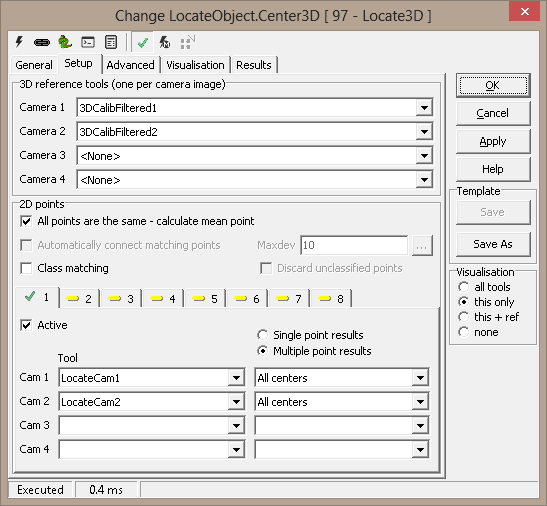Factor for calculating deviation

There are two deviation measures calculated for each point:

• The first (aka. Deviation or 3D Deviation) measures how well the observed points coincide in 3D space. A 3D point is found as the intersection of two or more 3D lines, one line from each camera. The lines are the "inverse projections" of the 2D points into space. When two lines do not meet exactly in space, the deviation is half of the shortest distance between them. i.e., by how much the point result deviates from the lines. When more that two cameras are used, the deviations are averaged. Deviation is given in physical coordinates and reflects 3D camera calibration
• The second (2D deviation) is calculated by mapping the found 3D point back to the camera image, and measuring the distance from this point to the incoming 2D point. A large error here could mean that the tools producing the 2D points have missed.

The weight percent lets you use either deviation measure or both:

• Weight percent deviation vs. 2D deviation -  100% means use 3D deviation only; 0% means 2D deviation only. Or you can select a combination. Generally this is sound, since the 2D and 3D measurements are in the same dimension (usually mm).

Constraints

• Max deviation for accepted point - acceptance criterion for each point (see Deviation above).
• Ignore camera points with deviation ratio over [dB] - ignore cameras where 3D deviation ratio to the best camera is over the threshold - measured in dB
• Ignore camera points with deviation over - used to remove "bad" points before estimating position in space
• Minimum number of active cameras - accept point only if at least this many cameras have a valid result

Constraints for mean point

This is active only if All points the same is checked on the Setup page. All found points are averaged and results taken from there.

• Max deviation (spread) for accepted point - acceptance criterion for the common (mean) point. The spread is calculated in 2D as the maximum distance from the incoming tool points to the mean point.
• Minimum number of accepted points - accept mean point only if at least this many points have a valid result.

Include in on-screen-description - requires description to be active under Visualisation

• Point number - (not available if All points the same is checked)
• 3D CoordinatesVisualisation

 AcceptedPoint Point that passes constraints FailDescription Description of a failed point FailedPoint Found point that fails constraints IncomingPoint Input points from other tools OKDescription Description of an accepted point

Results

 Point[1-8].x X position for found 3D point Point[1-8].y Y position for found 3D point Point[1-8].z Z position for found 3D point Deviation[1-8] Point deviation Cameras used[1-8] Number of used cameras for point Cameras in use[1-8] Python tuple of the cameras used to generate the result Accepted[1-8] Constraints result (0=fail, 1=accepted) Mean point (.x,.y,.z) Mean of found points (only for all points are the same) Spread Max distance from single point to the mean Active points Count of active input points Accepted points Count of points accepted and reported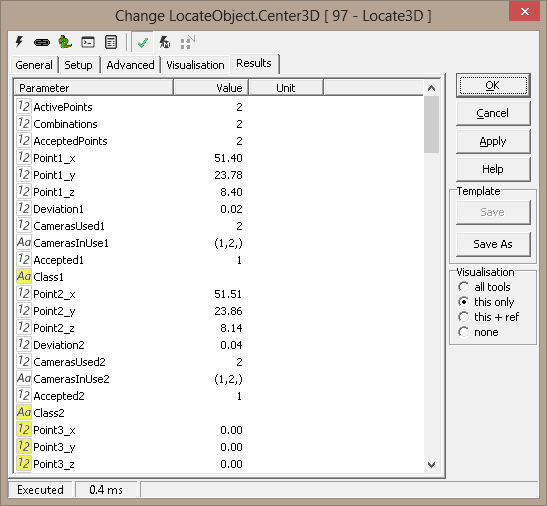Example 1: Measure Distance Between Points

```import math

def Distance3D (x1,y1,z1,x2,y2,z2):
return math.sqrt((x1-x2)*(x1-x2) + (y1-y2)*(y1-y2) + (z1-z2)*(z1-z2))

def GetPoint3D(name):
x = GetFloatValue(name+'_x')
y = GetFloatValue(name+'_y')
z = GetFloatValue(name+'_z')
return x,y,z

def SetPoint3D(name,x,y,z):
SetFloatValue(name+'_x',x)
SetFloatValue(name+'_y',y)
SetFloatValue(name+'_z',z)

x1,y1,z1 = GetPoint3D('Points3D1.Point1')
x2,y2,z2 = GetPoint3D('Points3D1.Point2')
x3,y3,z3 = GetPoint3D('Points3D1.Point3')

width = Distance3D(x1,y1,z1,x2,y2,z2)
height = Distance3D(x1,y1,z1,x3,y3,z3)

print 'w,h -',width,height```

 Scorpion Vision Version XII : Build 646 - Date: 20170225 Scorpion Vision Software® is a registered trademark of Tordivel AS. Copyright © 2000 - 2017 Tordivel AS.